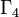# Nilpotency class and order need not determine conjugacy class size statistics for groups of prime-fifth order

## Statement

Let$p$ be a prime number. It is possible to have two groups$P_1$ and$P_2$, both of order$p^5$, such that$P_1$ and$P_2$ have the same nilpotency class but have different conjugacy class size statistics.

## Proof

### Case$p = 2$

Further information: Element structure of groups of order 32#Conjugacy class sizes

For groups of order 32, there are three different Hall-Senior families of groups,$\Gamma_2$,$\Gamma_4$, and$\Gamma_5$, all of which comprise groups of nilpotency class two, but with the groups in each family having different conjugacy class size statistics from each other:

• The family$\Gamma_2$ contains 15 groups, such as direct product of D8 and V4, and all groups in this family have conjugacy class sizes as follows: 8 conjugacy classes of size 1, 12 conjugacy classes of size 2.
• The family$\Gamma_4$ contains 9 groups, such as generalized dihedral group for direct product of Z4 and Z4, and all groups in this family have conjugacy class sizes as follows: 4 conjugacy classes of size 1, 6 conjugacy classes of size 2, 4 conjugacy classes of size 4.
• The family$\Gamma_5$ contains 2 groups, namely, the two extraspecial groups of order 32 (inner holomorph of D8 and central product of D8 and Q8) and both have conjugacy class sizes as follows: 2 conjugacy classes of size 1, 15 conjugacy classes of size 2.